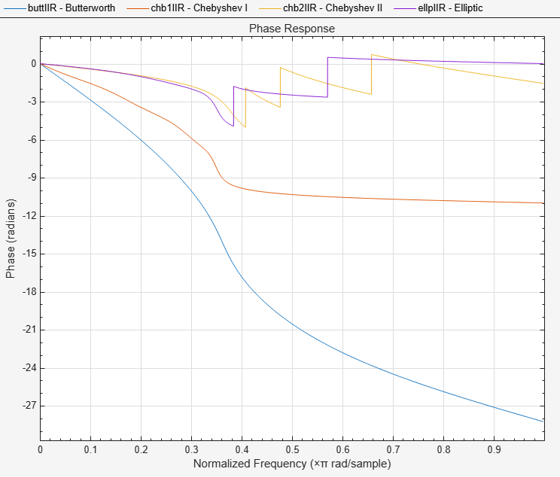Documentation

# islinphase

Determine whether filter has linear phase

## Syntax

flag = islinphase(b,a) flag = islinphase(sos) flag = islinphase(d) flag = islinphase(...,tol) flag = islinphase(hs,...) flag = islinphase(hs,'Arithmetic',arithtype) 

## Description

flag = islinphase(b,a) returns a logical output, flag, equal to true if the filter coefficients in b and a define a linear phase filter. flag is equal to false if the filter does not have linear phase.

flag = islinphase(sos) returns true if the filter specified by second order sections matrix, sos, has linear phase. sos is a K-by-6 matrix, where the number of sections, K, must be greater than or equal to 2. Each row of sos corresponds to the coefficients of a second order (biquad) filter. The ith row of the sos matrix corresponds to [bi(1) bi(2) bi(3) ai(1) ai(2) ai(3)].

flag = islinphase(d) returns true if the digital filter, d, has linear phase. Use designfilt to generate d based on frequency-response specifications.

flag = islinphase(...,tol) uses the tolerance, tol, to determine when two numbers are close enough to be considered equal. If not specified, tol, defaults to eps^(2/3).

flag = islinphase(hs,...) determines whether the filter System object™, hs, has linear phase. You must have the DSP System Toolbox™ to use islinphase with a System object.

flag = islinphase(hs,'Arithmetic',arithtype) analyzes the filter System object hs based on the specified arithtype. arithtype can be one of 'double', 'single', or 'fixed'. When you specify 'double' or 'single', the function performs double- or single-precision analysis. When you specify 'fixed' , the arithmetic changes depending on the setting of the CoefficientDataType property and whether the System object is locked or unlocked. You must have the DSP System Toolbox to use islinphase with a System object.

When you do not specify the arithmetic for non-CIC structures, the function uses double-precision arithmetic if the filter System object is in an unlocked state. If the System object is locked, the function performs analysis based on the locked input data type. CIC structures only support fixed-point arithmetic.

## Examples

collapse all

Use the window method to design a tenth-order lowpass FIR filter with normalized cutoff frequency 0.55. Verify that the filter has linear phase.

firSpecs = fdesign.lowpass('N,Fc',10,0.55); lpFIR = design(firSpecs,'window','SystemObject',true); flag = islinphase(lpFIR)
flag = logical 1 
[phs,w] = phasez(lpFIR); plot(w/pi,phs) xlabel('Frequency \omega/\pi') ylabel('Phase')IIR filters in general do not have linear phase. Verify the statement by constructing eighth-order Butterworth, Chebyshev, and elliptic filters with similar specifications.

ord = 4; Wp = 0.35; Wst = 0.4; atten = 20; rippl = 1; buttSpecs = fdesign.lowpass('Fp,Fst,Ap,Ast',Wp,Wst,rippl,atten); buttIIR = design(buttSpecs,'butter','SystemObject',true); chb1Specs = fdesign.lowpass('Fp,Fst,Ap,Ast',Wp,Wst,rippl,atten); chb1IIR = design(chb1Specs,'cheby1','SystemObject',true); chb2Specs = fdesign.lowpass('Fp,Fst,Ap,Ast',Wp,Wst,rippl,atten); chb2IIR = design(chb2Specs,'cheby2','SystemObject',true); ellpSpecs = fdesign.lowpass('Fp,Fst,Ap,Ast',Wp,Wst,rippl,atten); ellpIIR = design(ellpSpecs,'ellip','SystemObject',true);

Plot the phase responses of the filters. Determine whether they have linear phase.

fv = fvtool(buttIIR,chb1IIR,chb2IIR,ellpIIR,'Analysis','phase'); legend(fv,'Butterworth','Chebyshev I','Chebyshev II','Elliptic')phs = [islinphase(buttIIR) islinphase(chb1IIR) ... islinphase(chb2IIR) islinphase(ellpIIR)]
phs = 1x4 logical array 0 0 0 0